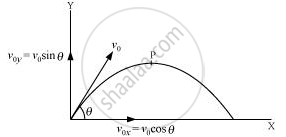# Show that for a Projectile the Angle Between the Velocity and the X-axis as a Function of Time is Given by - Physics

Show that for a projectile the angle between the velocity and the x-axis as a function of time is given by

theta(t) =tan^(-1) ((v_(0y) - "gt")/v_(o x))

#### Solution 1

Let v_(ox) and v_(oy) respectively be the initial components of the velocity of the projectile along horizontal (x) and vertical (y) directions.

Let v_x and v_y respectively be the horizontal and vertical components of velocity at a point P.Time taken by the projectile to reach point P = t

Applying the first equation of motion along the vertical and horizontal directions, we get:

v_y = v_(oy) = "gt"

And v_x   =v_(ox)

:. tan theta = v_y/v_x= (v_(oy) - "gt")/v_(ox)

theta = tan^(-1)((v_(oy) - "gt")/(v_(o x)))

#### Solution 2

Let the projectile be fired at an angle theta with x-axis.

As theta depend on t, theta(t) at any instance

tan theta(t) = v_y/v_x =(v_(oy) - "gt")/(v_(o x))

(Since v_y = v_(oy) - "gt" and v_x = v_(o x))

=> theta(t) = tan^(-1)((v_o - "gt")/v_(o x))

Concept: Projectile Motion
Is there an error in this question or solution?
Chapter 4: Motion in a Plane - Exercises [Page 88]

#### APPEARS IN

NCERT Class 11 Physics
Chapter 4 Motion in a Plane
Exercises | Q 32.1 | Page 88
Share